# USB Voltage And Current Monitor Using MBDT, Freemaster And MPC5744P-DEVKIT

cancel
Showing results for
Did you mean:

No ratings

# 1. Introduction

The scope of this article is to show how easy it is to use MBDT, FreeMASTER and some knowledge in electronics to create a simple and useful project: monitor USB voltage and current consumption.

I used this setup it to monitor USB voltage and current while my smartphone is charging, but it can be used in other purposes too, like monitoring power consumption of some devices connected on USB charger/power bank/PC, or it can be used to find some charging issues, or maybe you just want to find out if your phone charger supports fast charging, or to characterize a power bank.

# 2. Overview

#### 2.1 Functional Description

This project can measure USB voltage and current by measuring USB voltage and a voltage drop across a resistor through which current flows from a USB power source to a USB load. The voltage given by current flow is amplified by an electronic circuit and sampled by MPC5744P-DEVKIT board through the ADC converter, then data is sent to PC and then are displayed through a FreeMASTER project as numerical values and as oscilloscope chart. The MPC5744P-DEVKIT board is programmed by MBDT.

To build this simple project, you need three components: Matlab with MBDT toolbox installed, FreeMASTER Run-Time Debugging Tool, a very simple electronic circuit, and an MPC5744P-DEVKIT

As can be seen in fig.1, the project can be split into two main parts: Hardware Setup and Software Setup.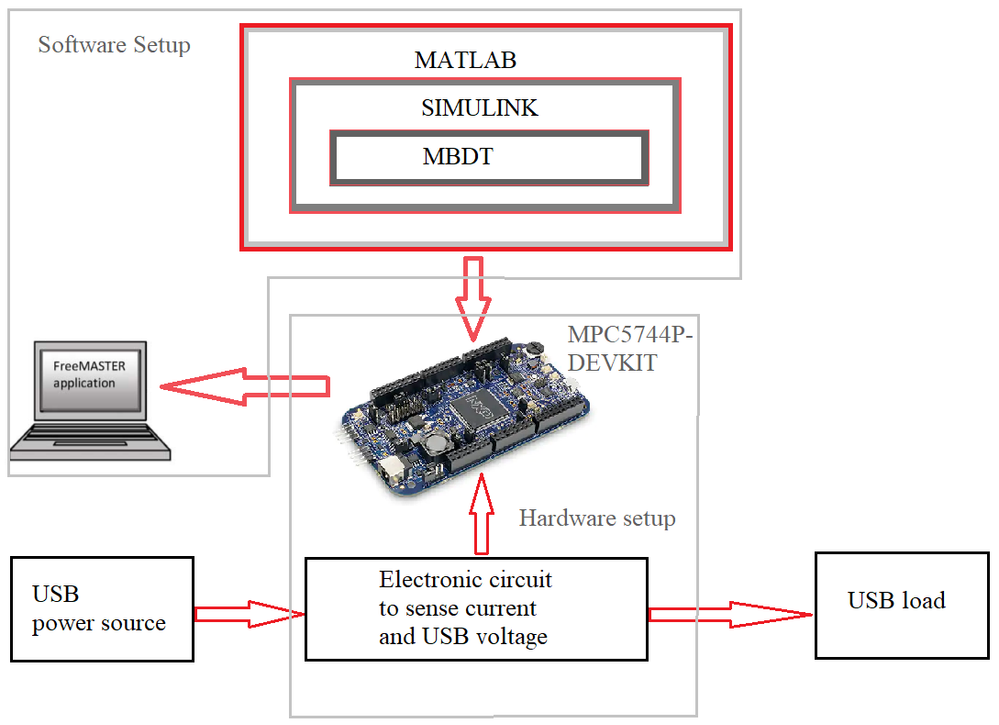Fig. 1: USB Voltage And Current Monitor block diagram

## 2.1 Hardware Setup

### Custom Electronic Board

The simplest way to measure current is to use a simple resistor connected in series with the load (USB output in our case). The Ohm law gives us a linear relationship between voltage and current in an electrical circuit:

U = R * I

U is the voltage (measured in volts V) drop across the resistor, R is the resistance of the resistor (measured in ohm Ω) and I is the current (measured in amps A) which we need to measure.

Most of the USB chargers can supply a maximum of 3A and most of the smartphones can drain less than 3A. Giving that I considered designing this circuit to measure around maximum 3A. Let suppose that we use a simple 1ohm resistor. If we do simple math using Ohm formula, then it can be seen that for 3A the voltage across our resistor (there) will be 3V (U = 1 * 3).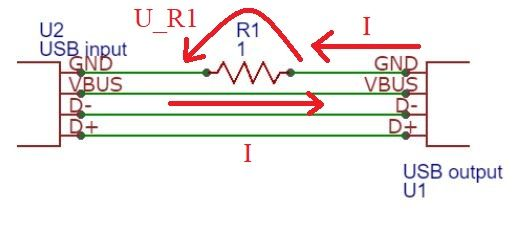Fig. 2: USB connectors and current "sensor"

In Fig. 2 on the left side is a USB input connector, on the right side is the output USB connector and both GND lines are connected through R1 current sensor. The current "I" will flow, as can be seen in Fig. 2, from USB input through VBUS  line to VBUS on USB output, than will flow through our load connected to USB output and then through GND on the same connector thru R1 and at least to GND on USB input connector. This current will create a voltage across R1 (UR1) equal with 3V (if we use 3 amps current and 1-ohm resistor).

One of USB standard charging it specifies that the lowest (standard) voltage it's 5V. In our case, using this 1 ohm resistor will cause 3V loss, then the voltage at USB outputs will be 2V (unacceptable). In most of the digital electronic circuits, when it's used 5 volts power, the voltage tolerance it's about 10% (0.5V) which means the voltage can be between 4.5V and 5.5V. Let's try to create a maximum voltage drop across this resistor about 3 times lower than the 0.5V, e.g.: 150mV. Then the current sensor resistor is R1 = 0.15V / 3A = 0.05ohm.

To measure this drop voltage we could use any ADC channel on MPC5744P-DEVKIT, but if the voltage reference of ADC is set to 5V, the resolution of ADC is 5V/4096 = 1.22mV, which means the equivalent ADC resolution corresponding to current measurement is about 24mA (1.22mV correspond to 24mA). If we consider electrical noise on USB connectors, it's very possible that the out signal will be "drowned" in electrical noise or the results will be not very satisfactory.

The solution to that problem is to "rescale" this 0V --> 0.15V range to 0V --> 5V. We can do that by using operational amplifier circuits.

I used what I found on the moment in my electronic components, an LM358 IC.  This IC is not the best, but for the demo, it's ok to be used.Fig. 3: USB current and voltage monitor

A few ideas of this electronic design:

• this IC contains two operational amplifiers,
• first amplifier coefficient is set thru R5 and R4 (34 amplification coefficient) and the signal is connected on his noninverting input,
• the lowest current that can be measured is approx. 0.7mA,
• the second amplifier is set as a buffer amplifier,
• note: if the maximum current used by the load is maximum 3A, the Zenner diode it's not necessary.

By using this amplifier, the range is rescaled to 0 --> 5.1V.

To measure the voltage at USB input we use R9 and R10 divider resistor and will use the formula U_R9 = U_USB * ( R9/(R9 + R10)). And by using for R9 10K and for R10 33K

the voltage measured by ADC for maximum USB standard charging is U_R9 = 20V * (10/(43)) = 4.65V.

This circuit has been developed on prototyping PCB. The outputs of this circuit are connected to two ADC (current signal to PTB13 and voltage signal to PTB14) channels of MPC5744P-DEVKIT and those can be found on CN1 connector ("USB voltage", "USB current" and GND).

### MPC5744P-DEVKIT Evaluation Board

The main scope of this devkit is to get current and voltage data from the custom board and to convert it to digital data and send it to the PC through the UART interface.

As can be seen in fig. 4, I used three wires to connect the custom electronic board to the devkit (two ADC and GND).

On MPC5744P-DEVKIT the ADC voltage reference must be set to 5V (jumper J19 --> 2-3) and the data will be sent to PC through UART (USB --> OpenSDA).

The MPC5744P-DEVKIT must be connected to PC thru USB cable. All other jumpers can be let in the default state.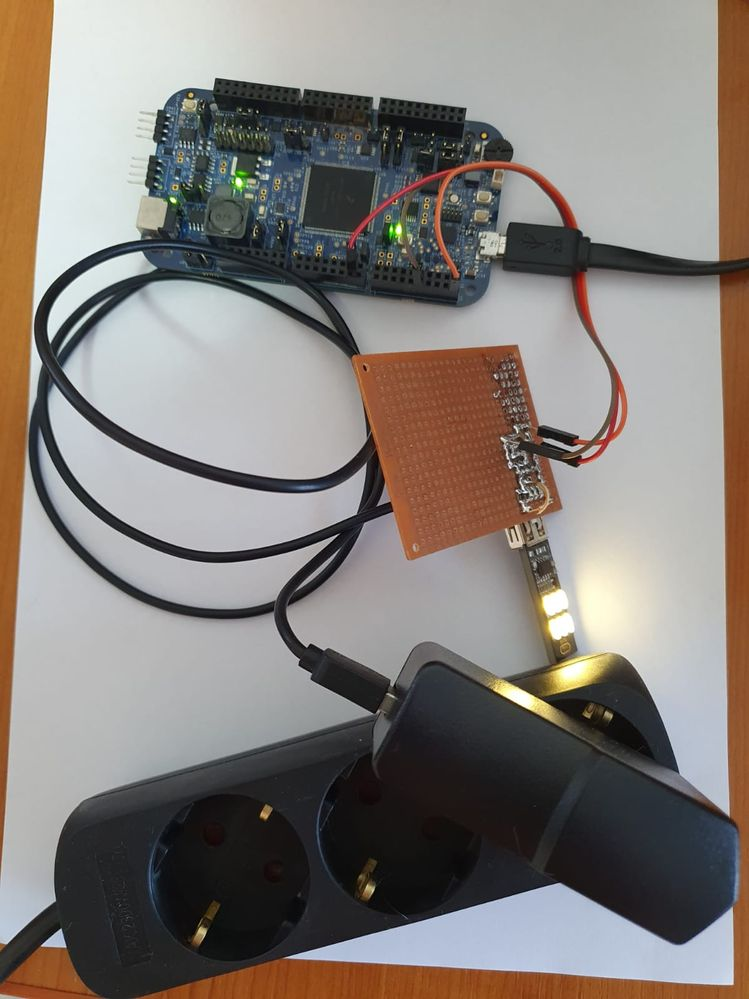Fig. 4: Hardware setup (load is a USB led light)

## 2.1 Software Setup

### MBDT Application Model

For software development, I used Matlab with the MBDT toolbox. The first step is to create a new Simulink project using MBDT. The project contains three main parts:

• configuration blocks
• variable declaration blocks
• main loop, where the values are taken from the output of ADC block, and after using some simple maths functions, resulted in values are stored in current and voltage variables.

After getting the values from ADC blocks, we must apply some math functions to reverse the effect created in the electronic circuit.

Part of the configuration blocks contain:

• MPC5744P configuration block,
• ADC configuration block, which has two channels are configured,
• FreeMASTER block used to observe the current, voltage, and other parameters over UART through the FreeMASTER protocol.

Part of the variables declaration is used to declare all variables used to get values from ADC peripheric, intermediate values, and final values.

Part of the main loop consists of simple mathematical functions that have the role to convert ADC values to current and voltage values.

The first thing executed is taking the ADC current and voltage values, then those values are converted to floating-point type the multiplied with a constant (ADC_Vref /ADC_resolution = 5/4095) which represent ADC voltage resolution. Then, for USB current value, the result must be divided with operational amplifier factor (34), after that, we could subtract from the result that operational amplifier input offset (if it is measured any relevant value) and the last thing to do to get the USB current is to convert from voltage to current using ohm law (U = R * I).

To get the USB voltage, the first step is similar to the USB current value, using the resistor divider formula we get the final value.

For using different opamp IC, if the non-inverting input is too high, it is recommended to be measured while the electric circuit is on and the value to be updated in the model (variable Current_offset).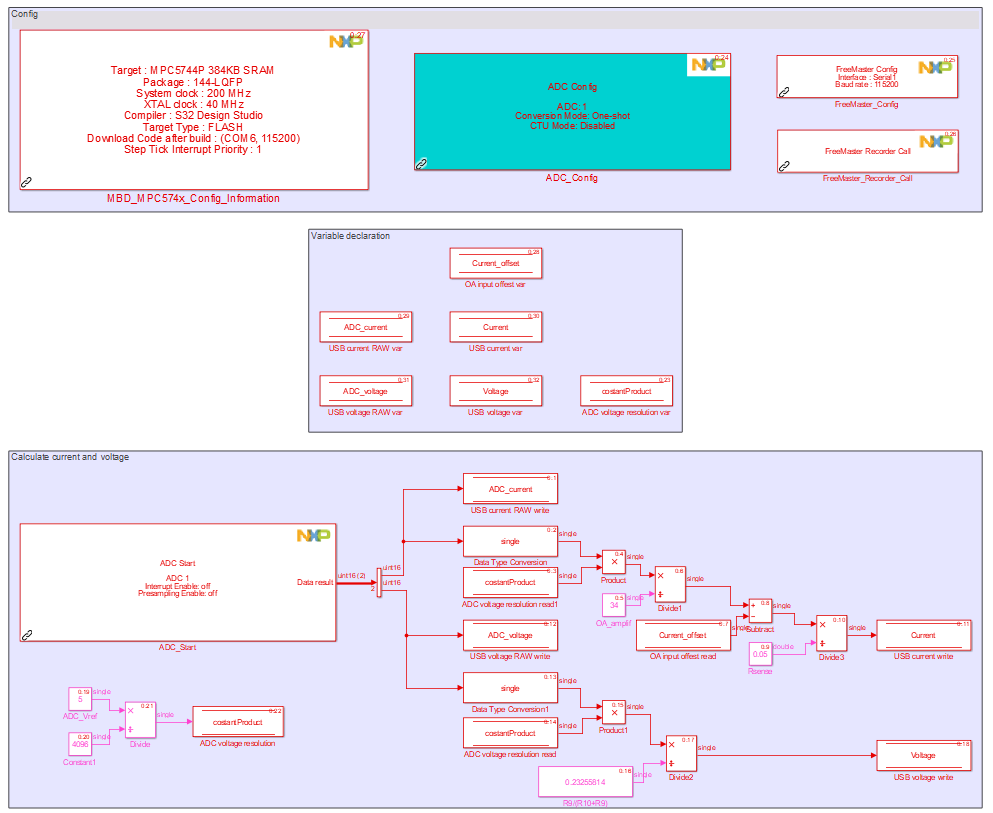Fig. 5: MBDT Application Model

### FreeMASTER project

To view data in real-time, the first thing is to open the FreeMASTER project and, select "Tools", then "Connection Wizard ...", then hit "Next" button, select the first option "Use the direct connection to an on-board USB port", then select the serial com port assigned to MPC5744P-DEVKIT and 115200 baud rate.

The USB current and voltage can be observed In the FreeMASTER project as a numerical value and as an oscilloscope view by selecting "RAW data" to plot raw ADC USB current and voltage values. To plot the real USB current and voltage values, in the Project Tree, please select "Voltage and current" oscilloscope.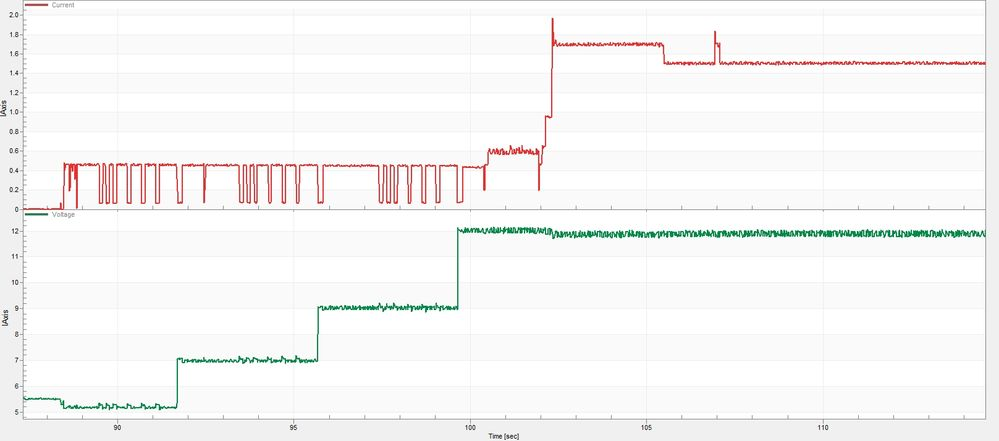Fig. 6: FreeMASTER USB current and voltage monitor

In Fig. 6 it can be seen current and voltage variations in time for fast charging transitions when my smartphone is connected to the original charger.

Version history
Revision #:
1 of 1
Last update:
‎04-22-2020 11:52 PM
Updated by: